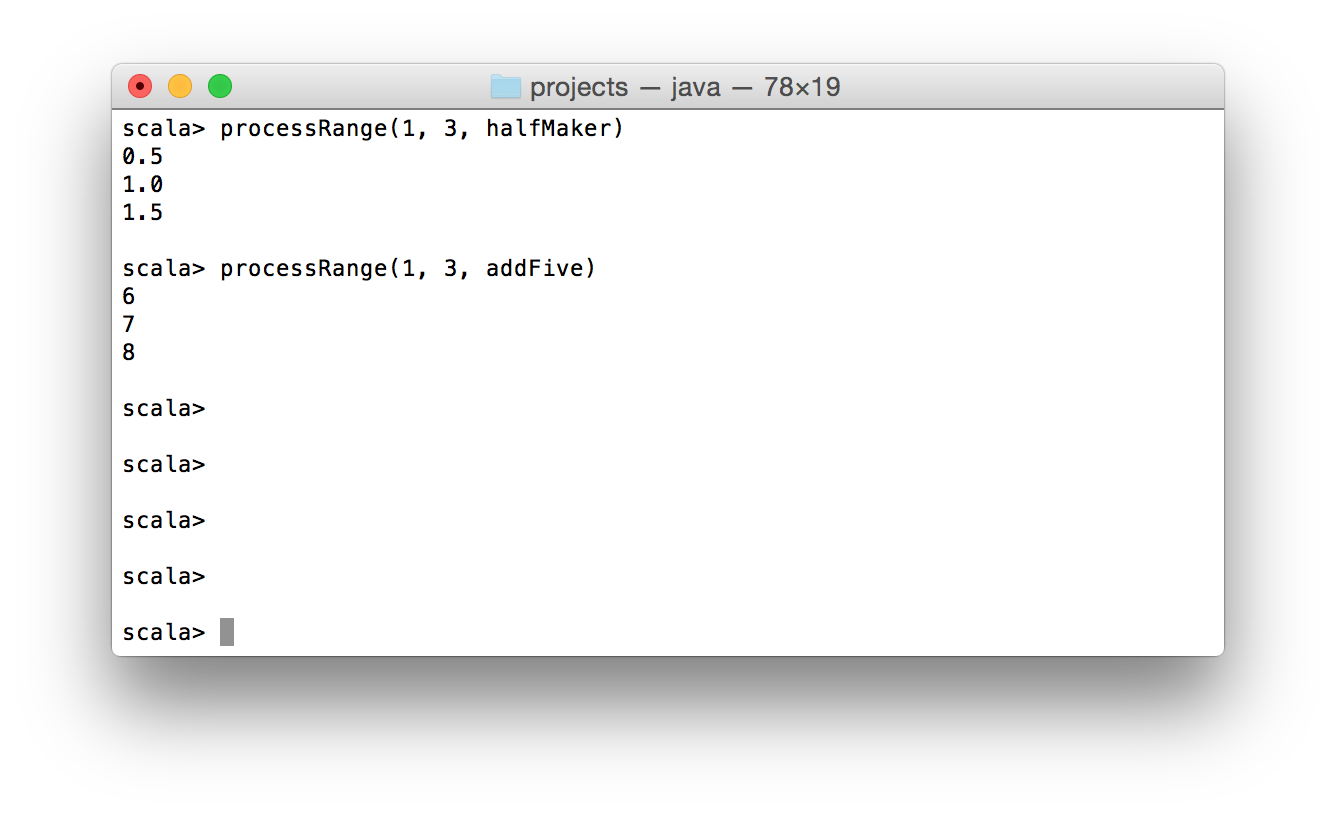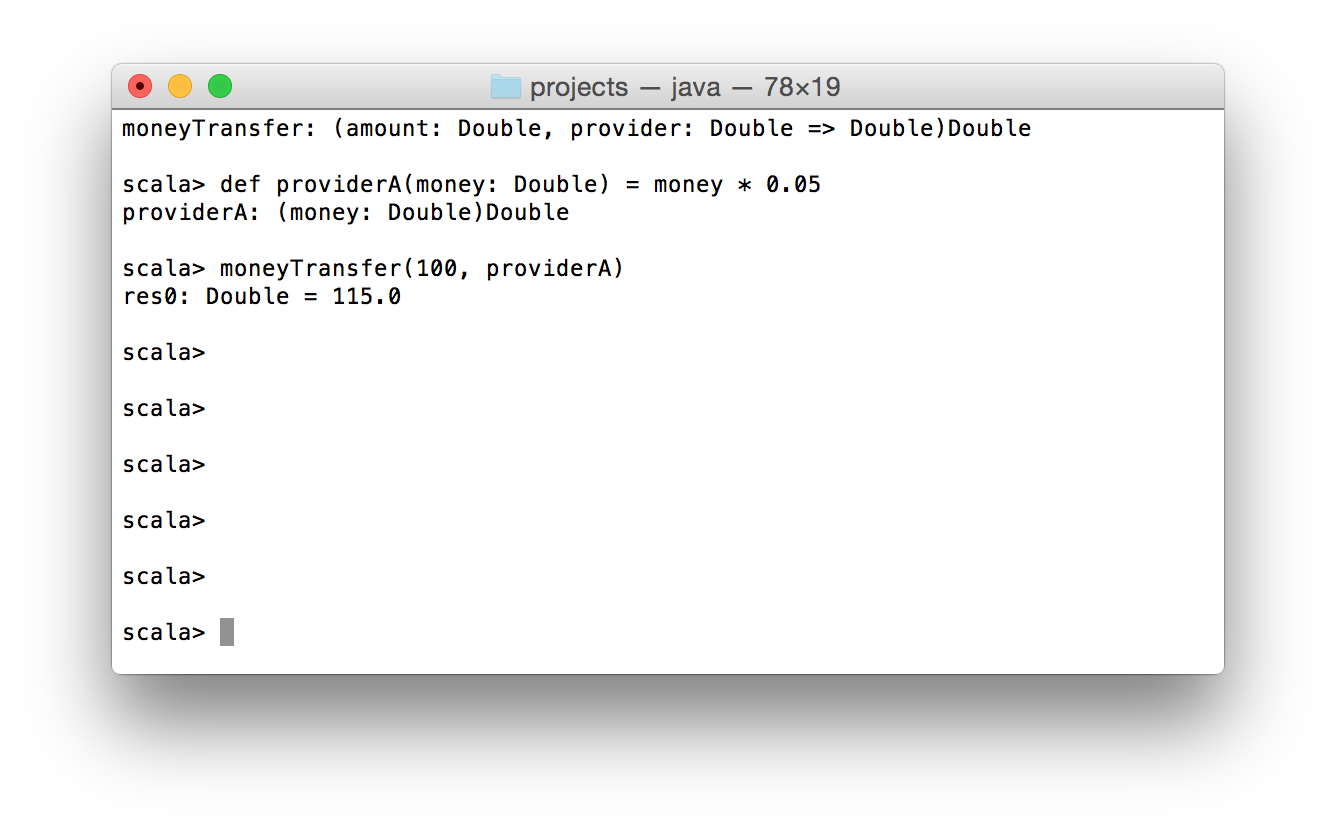Refcards Trend Reports Events Over 2 million developers have joined DZone. Join Today! Thanks for visiting DZone today,
Register Now

# Scala: Higher-Order and Anonymous Functions

### Check out this look at Scala high-order and anonymous functions, with a focus on funciton as parameter, the anonymous function, and function as return value.

· Tutorial
Save
17.69K Views

now after we have considered basics of scala functions , we can make the next step in more complex use cases functional programming. since functions in scala are objects we can pass them as arguments in other functions, also we can return them as a result. in this article, i’m going to examine multiple examples of higher-order functions .

what functions? a function which accepts another function as arguments or returns a function is a higher-order function. it sounds like a madness if you have a previous experience with object-oriented programming, but after the reading, you’d definitely understand how it works.

## function as parameter

first, let’s do what we already know how to do – declare a simple function.

``````def halfmaker(value: int): double = value.todouble/2
``````

this is a small function with a trivial logic: accept ``` int ``` value and return a half of it in a ``` double ``` type.

what about one more simple function?

``````def addfive(value: int): int = value+5
``````

``` addfive ``` function even more trivial than the ``` halfmaker ``` . it accepts ``` int ``` value and adds to it 5.

what is common in these two functions? they both accept ``` int ``` parameter and return an ``` anyval ``` value. so in general, we can describe these functions by the following signature ``` int => anyval ``` .

now let’s declare a function which has a function argument among other parameters.

``````def processrange(start: int, finish: int, processor: int => anyval): unit = {
for (i <- start to finish)
println(processor(i))
}
``````

as you see ``` processrange ``` for each ``` int ``` value between the ``` start ``` and ``` finish ``` , applies the ``` processor ``` function and prints a result. the type of the processor parameter is ``` int => anyval ``` . it matches the signatures of two first functions which we declared earlier in the article.

here is a demonstration of this code in action:of course, you need to declare all of the functions before their usage. a function passed as an argument to another function is a callback function .

let’s continue with one more example of callback functions in scala.

``````def moneytransfer(amount: double, providerfee: double => double): double = {
amount + 10 + providerfee(amount)
}

def providera(money: double) = money * 0.05

println(moneytransfer(100, providera))
//the output is 115.0
``````

this code isn’t good from the functional point of view, it doesn’t check that the ``` amount ``` is positive and more than 10 etc, but this example is just for demonstration of a callback function.

so let’s assume that we have an application which works with money transfers. we are a service provider. if people want to send money, they come to us and we give them an interface for this. in our turn, we use already existing services for this, e.g. provider a, provider b etc. our fee is \$ 10. depending on the provider, an extra charge occurs and they vary from provider to provider.

the ``` moneytransfer ``` function accepts two arguments: ``` amount ``` – amount of money which customer wants to transfer and ``` providerfee ``` is a callback function with signature ``` double => double ``` . the purpose of ``` moneytransfer ``` function is to calculate total which customer needs to provide in order to make a transfer.by passing a function as the argument you inject an extra logic inside of the higher-order function. so it’s very powerful mechanism to make you code more flexible and efficient. in the same time, you can complicate a simple code by redundant callbacks usage.

## anonymous function

sometimes you don’t need to declare a function before its usage because it needs to be used just in one place. for this case, anonymous functions suit well. let’s use one of the previous examples for demonstration:

``````def moneytransfer(amount: double, providerfee: double => double): double = {
amount + 10 + providerfee(amount)
}

println(moneytransfer(100, moneytransfer(250, m => m / 10)))
//the output is 285.0
``````

so instead of declaring a regular function with signature ``` double => double ``` , we directly passed the anonymous function ``` m => m / 10 ``` . the type of ``` m ``` is omitted because it can be defined based on the context where the anonymous function is invoked.

``````//also valid
moneytransfer(100, moneytransfer(250, (m: double) => m / 10))
``````

sometimes an anonymous function is called lambda function . so you can use this name as an alias for an anonymous function.

## function as return value

the rareest case of higher-order functions is when a function returns another function. when it’s handy? if to been honest, i still don’t know good use cases for this. but scala gives you the chance to do this.

so let’s consider the following example:

``````def getstrategy(enoughenergy: boolean) = {
if (enoughenergy)
(energy: double) => "we are going to attack with damage "+energy
else
(energy: double) => "we are going to reflect damage "+energy/2
}

val returnedfunction = getstrategy(true)

returnedfunction(15.0)
//we are going to attack with damage 15.0
``````

that’s how a function can be returned as a result.

## summary

now you know how to use higher-order functions. maybe it’s hard to start to use them almost instantly after the reading of this article, but i believe that you will be able to read and understand a code where higher-order functions are used. the easiest part of the blog post is about anonymous functions. they are compact and behave like normal functions, except that circumstance that they don’t have names.

Published at DZone with permission of Alexey Zvolinskiy, DZone MVB.

Opinions expressed by DZone contributors are their own.